# Depreciation

Guide to Understanding Depreciation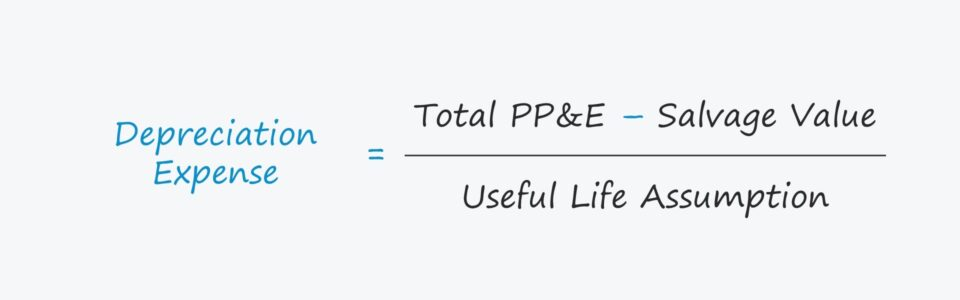## How to Calculate Depreciation?

Depreciation is required under U.S. GAAP accrual accounting due to the matching principle, which attempts to recognize expenses in the same period as when the coinciding revenue was generated.

In theory, this is a more accurate representation of the operational performance of the company, since the capital expenditure required to purchase the fixed asset is recognized across time span wherein it is generating revenue.

The concept of depreciation is an important consideration in order to understand the true cash flow profile of a company since it is a non-cash expense and can often be affected by discretionary assumptions by the company (i.e. determining the useful life).

• Non-Cash Expense: Depreciation is added back on the cash flow statement (CFS) as it is a non-cash expense – this means that there was no actual cash outflow despite depreciation being classified as an expense on the income statement and reducing earnings.
• Tax Shield: While depreciation is treated as a non-cash expense and added back on the cash flow statement, the expense reduces the tax burden for the period since it is tax-deductible.
• Net Income: The recognition of depreciation on the income statement results in some “noise” when evaluating the net income as recorded on the income statement and is why the cash flow statement is also necessary to evaluate a company’s performance.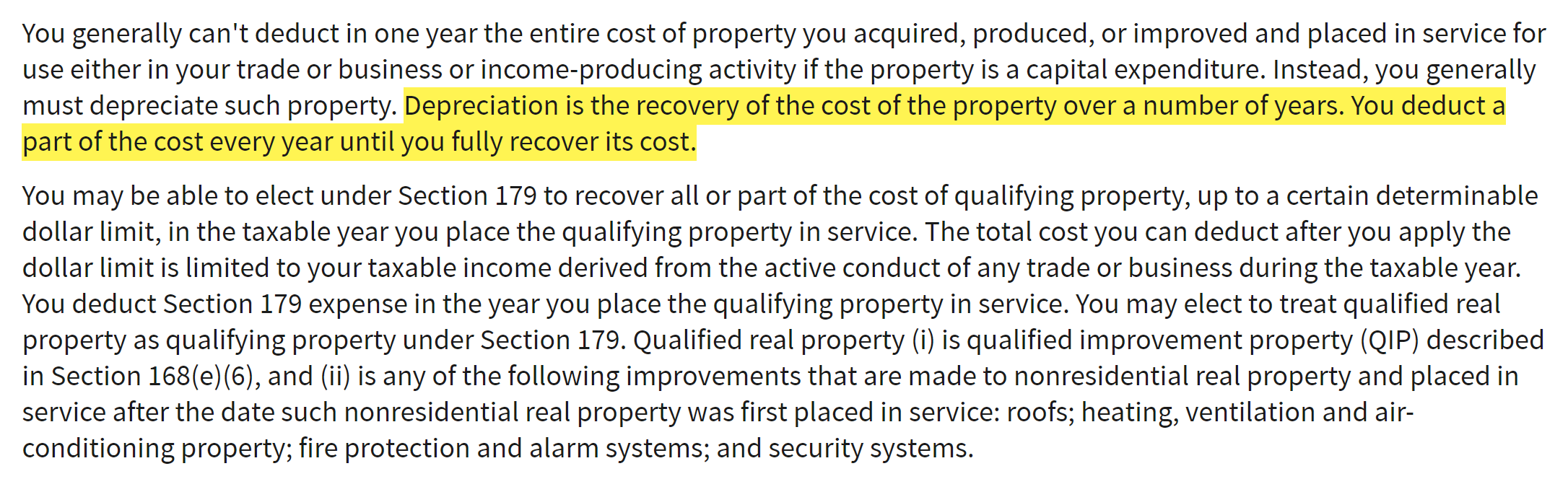IRS Topic No. 704 (Source: IRS)

## Is Depreciation an Operating Expense?

The depreciation expense, despite being a non-cash item, will be recognized and embedded within either the cost of goods sold (COGS) or the operating expenses line on the income statement.

As such, the recognition of depreciation on the income statement reduces taxable income, which leads to lower net income (i.e., the “bottom line”).

It is rather uncommon for companies to report depreciation as a separate expense on their income statement. Thus, the cash flow statement (CFS) and footnotes are recommended financial filings to obtain the value of a company’s depreciation expense.

## Depreciation Formula

The depreciation expense is scheduled over the number of years corresponding to the useful life of the respective asset.

Depreciation Expense = (Total PP&E Cost Salvage Value) / Useful Life Assumption
• Depreciation Expense: The depreciation expense represents the allocation of the one-time capital expenditure cash outflow throughout the useful life of the fixed asset – in an effort to decrease the value of the asset on the balance sheet as it helps produce revenue for the company.
• Salvage Value: The salvage value is defined as the value of the asset at the end of its useful life. Practically, salvage value can be thought of as the amount at which a company can sell the old asset at the end of its useful life.
• Useful Life Assumption: Once purchased, PP&E is a non-current (i.e. long-term) asset that continues to provide benefits to the company for the duration of its useful life, which is an estimate of how long the asset will continue to be used and be of service to the company.

## Quick Depreciation Calculation Example

If a manufacturing company were to purchase \$100k of PP&E with a useful life estimation of 5 years, then the depreciation expense would be \$20k each year under straight-line depreciation.

Therefore, \$100k in PP&E was purchased at the end of the initial period (Year 0) and the value of the purchased PP&E on the balance sheet decreases by \$20k each year until it reaches zero by the end of its useful life (Year 5).

• PP&E Purchase (Capex) = \$100k
• Useful Life Assumption = 5 Years
• Salvage Value (Residual) = \$0
• Annual Depreciation = \$100k / 5 Years = \$20k

Assuming the company pays for the PP&E in all cash, that \$100k in cash is now out the door, no matter what, but the income statement will state otherwise to abide by accrual accounting standards. This “smooths out” the company’s income statement so that rather than showing the \$100k expense entirely this year, that outflow is effectively being spread out over 5 years as depreciation.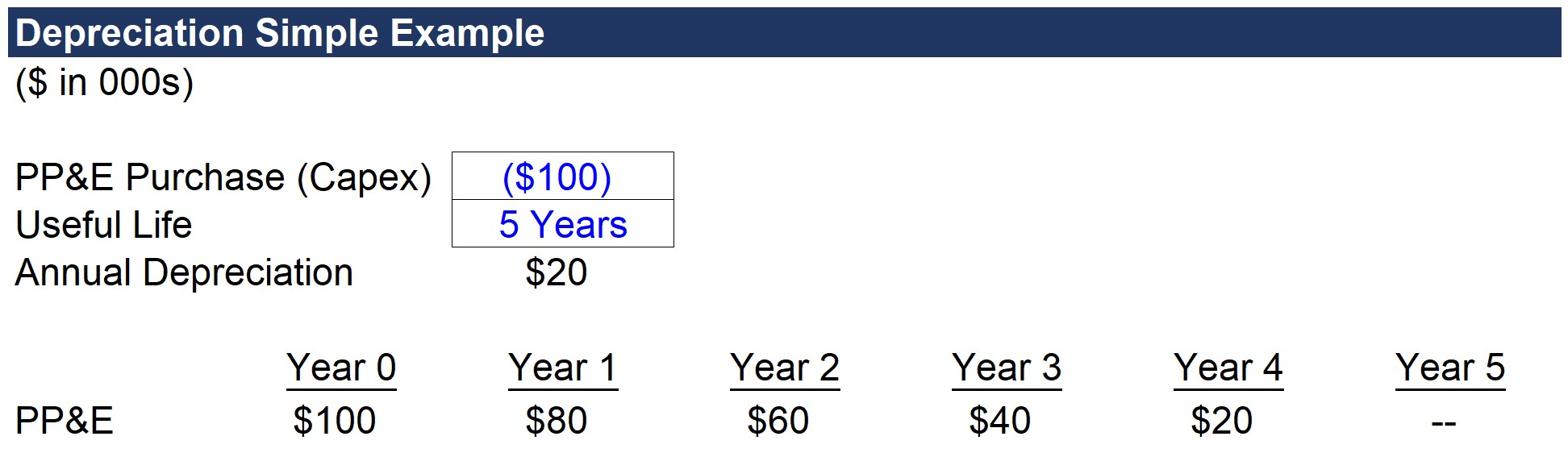## What is the Difference Between Straight-Line vs. Accelerated Depreciation?

There are various depreciation methodologies, but the most common type is called “straight-line” depreciation.

• Straight Line Method: Under the straight line method of depreciation, the carrying value of PP&E on the balance sheet is decreased evenly per year until the residual value has diminished to zero. The majority of companies use a salvage value assumption in which the remaining value of the asset becomes zero by the end of the useful life. In doing so, the depreciation expense each year will be higher, and the depreciation tax benefits are fully realized if the salvage value is assumed to be zero (and this is the common assumption used in straight-line depreciation).
• Accelerated Methods: There are other approaches to calculating depreciation, most notably accelerated accounting, which depreciates the asset faster in earlier years. In turn, this methodology lowers net income more in early years as compared to later years. However, considering how much publicly traded companies care about their net income and earnings per share (EPS) figures – most opt for straight-line depreciation.

At the end of the day, the cumulative depreciation amount is exactly the same, as is the timing of the actual cash outflow, but the difference lies in the net income and EPS impact for reporting purposes.

But in practice, most companies prefer straight-line depreciation for GAAP reporting purposes because lower depreciation will be recorded in the earlier years of the asset’s useful life than under accelerated depreciation. As a result, companies using straight-line depreciation will show higher net income and EPS in the initial years.

Under the accelerated depreciation method, net income and EPS would be lower in the earlier periods and then be higher relative to straight-line depreciation in later years – however, companies tend to prioritize near-term earnings performance.

The assumption behind accelerated depreciation is that the asset drops more of its value in the earlier stages of its lifecycle, allowing for more deductions earlier on.

The accelerated approach eventually begins to show less depreciation on the income statement further into the asset’s useful life, but to reiterate, companies still prefer straight-line depreciation because of the timing (i.e., avoid missing EPS figures on earnings releases).

## Accounting Interview Question: Depreciation 3-Statement Impact

To ensure you understand the non-cash feature of depreciation, we will go through this classic accounting interview question:

Q. “How would a \$10 increase in depreciation impact the three financial statements?”

• Income Statement: If depreciation increases by \$10, operating income (EBIT) would decrease by \$10. Assuming a 30% tax rate, net income would decline by \$7.
• Cash Flow Statement: At the top of the cash flow statement, net income is down by \$7, but recall the \$10 depreciation is a non-cash expense and therefore added back. The net impact on the ending cash balance is a \$3 increase, which comes from the tax-deductibility of depreciation (i.e., the \$10 in depreciation x 30% tax rate).
• Balance Sheet: PP&E will decrease by \$10 from the depreciation, while cash will be up by \$3 on the assets side. On the contrasting Liabilities & Equity side, the \$7 reduction in net income flows through to retained earnings. The balance sheet remains balanced since both sides went down by \$7.

The key takeaway is that depreciation, despite being a non-cash expense, reduces taxable income and has a positive impact on the ending cash balance.

## Depreciation to Capex Ratio Analysis

The “quick and dirty” method for projecting capital expenditures (Capex) and depreciation is as follows:

1. Capex: % of Revenue
2. Annual Depreciation: % of Capex (or Revenue)

Capital expenditures are directly tied to “top line” revenue growth – and depreciation is the reduction of the PP&E purchase value (i.e., expensing of Capex).

Capex can be forecasted as a percentage of revenue using historical data as a reference point. In addition to following historical trends, management guidance and industry averages should also be referenced as a guide for forecasting Capex.

In turn, depreciation can be projected as a percentage of Capex (or as a percentage of revenue, with depreciation as a % of Capex calculated separately as a sanity check).

For mature businesses experiencing low, stagnating or declining growth, the depreciation/Capex ratio converges near 100%, as the majority of total Capex is related to maintenance CapEx.

• Growth Capex: Companies experiencing high growth usually will have capex as a relatively greater percentage of their revenues. In short, the more a company has spent on Capex in recent years, the more depreciation the company incurs in its near-term future. High-growth companies tend to spend heavily on growth capex (i.e., optional expenditures to fund growth and expansion plans), and therefore usually exhibit depreciation/CapEx ratios that far exceed 100%.
• Maintenance Capex: On the other hand, the capex of low-growth companies will consist of a smaller percentage of revenue, with most of it being maintenance capex, which refers to the routine spending required for operations to continue (e.g., to replace equipment, perform refurbishments). But if the company is still continuously purchasing fixed assets, it should require more time for the depreciation/Capex ratio to converge closer to 100% (i.e., until capex spend is scaled back).

## Depreciation Waterfall Schedule Build

Another method to project a company’s depreciation expense is to build out a PP&E schedule based on the company’s existing PP&E and incremental PP&E purchases.

Under this approach, the average remaining useful life for existing PP&E and useful life assumptions by management (or a rough approximation) is necessary for projecting new CapEx.

While more technical and complex, the waterfall approach typically does not yield a substantially differing result compared to projecting Capex as a percentage of revenue and depreciation as a percentage of Capex.

For a complete depreciation waterfall schedule to be put together, more data from the company would be required to track the PP&E currently in use and the remaining useful life of each. Additionally, management plans for future CapEx spending and the approximate useful life assumptions for each new purchase are necessary.

If the data is readily accessible (e.g., a portfolio company of a private equity firm), then this granular approach would actually be feasible, as well as be more informative than the simple percentage-based projection approach.

But in the absence of such data, the number of assumptions required based on approximations rather than internal company information makes the method ultimately be less credible.

## Depreciation Calculator

Now, we will go through a depreciation waterfall schedule forecast build. Fill out the form below to download the template that goes along with our step-by-step tutorial:Submitting ...

## 1. Revenue and Capex Projection

In our hypothetical scenario, the company is projected to have \$10mm in revenue in the first year of the forecast, 2021. The revenue growth rate will decrease by 1.0% each year until reaching 3.0% in 2025.

CapEx as a percentage of revenue is 3.0% in 2021 and will subsequently decrease by 0.1% each year as the company continues to mature and growth decreases.

By 2025, CapEx as a percentage of revenue will be 2.6%.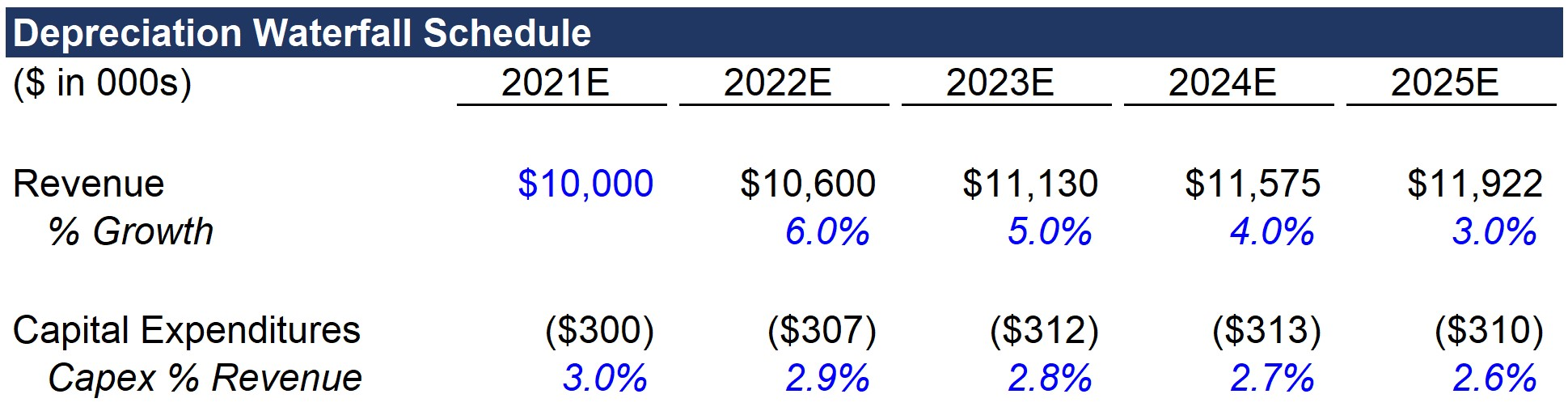Note that for purposes of simplicity, we are only projecting the incremental new capex.

In a full depreciation schedule, the depreciation for old PP&E and new PP&E would need to be separated out and added together.

## 2. Depreciation Waterfall Schedule Build in Excel

For the depreciation schedule, we will use the “OFFSET” function in Excel to grab the CapEx figures for each year.

While not overly useful for our simplistic model, it can help conserve time in more complex builds at the per-asset level. Regarding the inputs for the formula:

1. The first input is the reference cell, which is the cell to the left of the CapEx amount
2. Next, zero is entered for the row since we want to remain on Line 19
3. Lastly, we link to the “Year X” cell on the left for the column input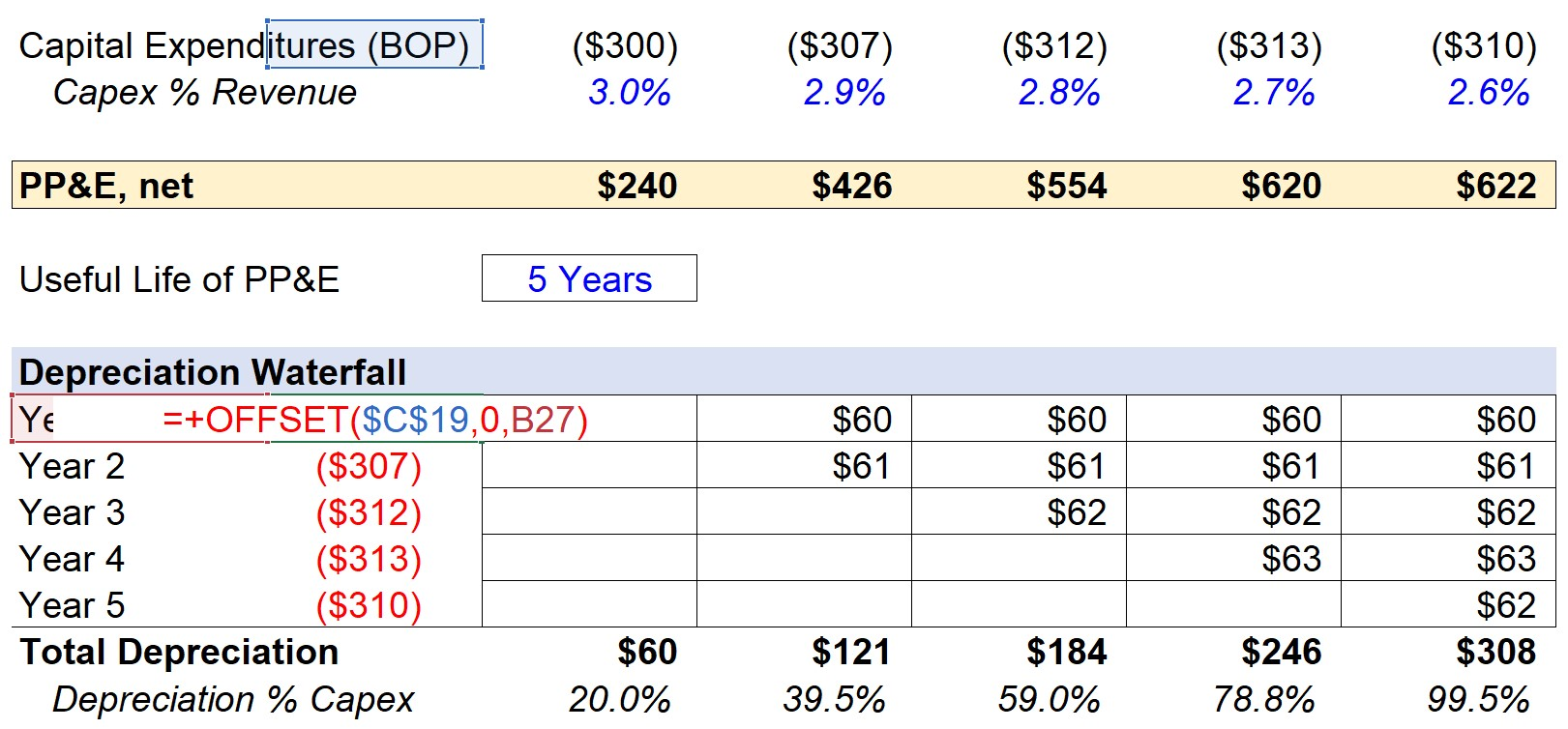In the first year, the PP&E balance in 2021 comes from the \$300k corresponding CapEx spend. Here, we are assuming the CapEx outflow is right at the beginning of the period (BOP) – and thus, the 2021 depreciation is \$300k in CapEx divided by the 5-year useful life assumption. This comes out to \$60k each year, which will remain constant until the salvage value reaches zero.

## 3. Depreciation Calculation Example

The formula for the annual depreciation is as follows.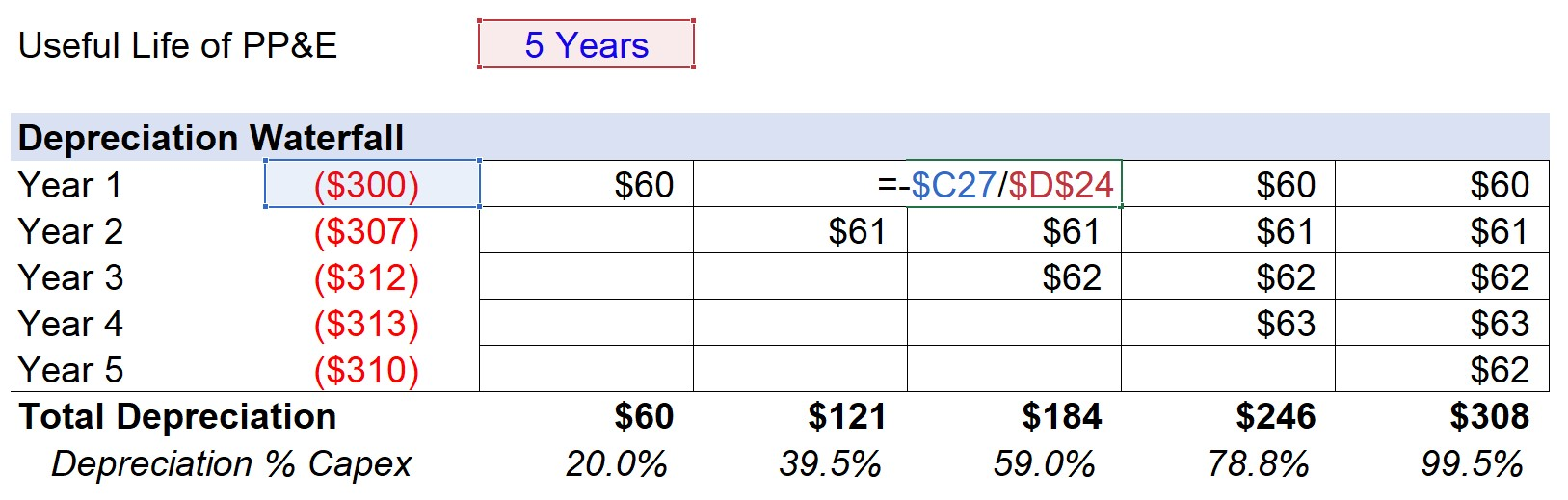The completed forecast for Year 1 has been highlighted in the screenshot below. As a sanity check, each of the numbers should be the same since we are using the straight-line approach in our example. And while not applicable for our projection, longer-term models should use a “MAX” function with the remaining salvage value to make sure it does not dip below zero.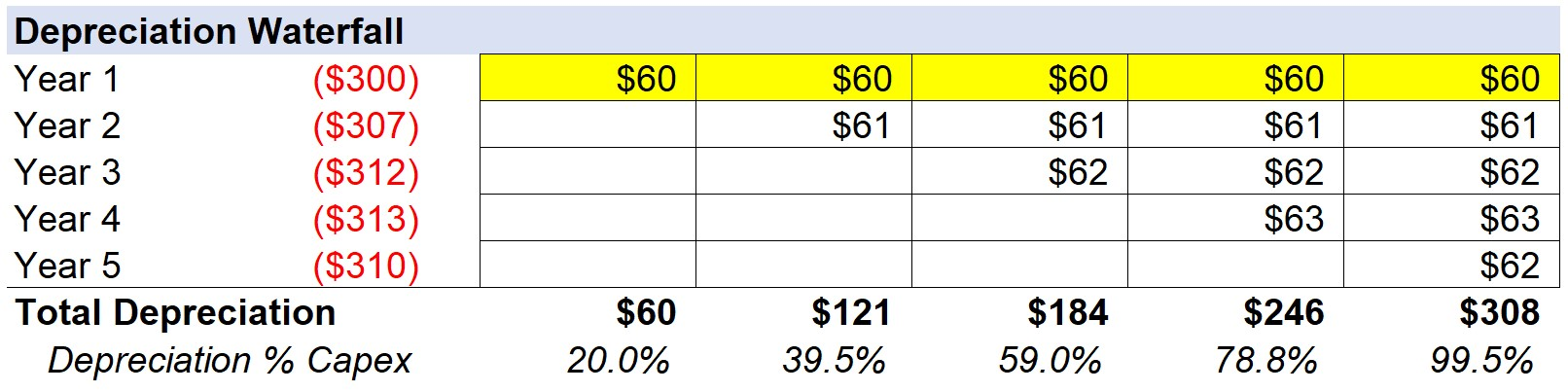Then, we can extend this formula and methodology for the remainder of the forecast. For 2022, the new CapEx is \$307k, which after dividing by 5 years, comes out to be about \$61k in annual depreciation.

Once repeated for all five years, the “Total Depreciation” line item sums up the depreciation amount for the current year and all previous periods to date. For example, the total depreciation for 2023 is comprised of the \$60k of depreciation from Year 1, \$61k of depreciation from Year 2, and then \$62k of depreciation from Year 3 – which comes out to \$184k in total.

Returning to the “PP&E, net” line item, the formula is the prior year’s PP&E balance, less capex, and less depreciation.

• Current Year PP&E = Prior Year PP&E – CapEx – Depreciation

Since Capex was input as a negative, the CapEx will increase the PP&E amount as intended (otherwise, the formula would have added Capex if the positive sign convention had been used).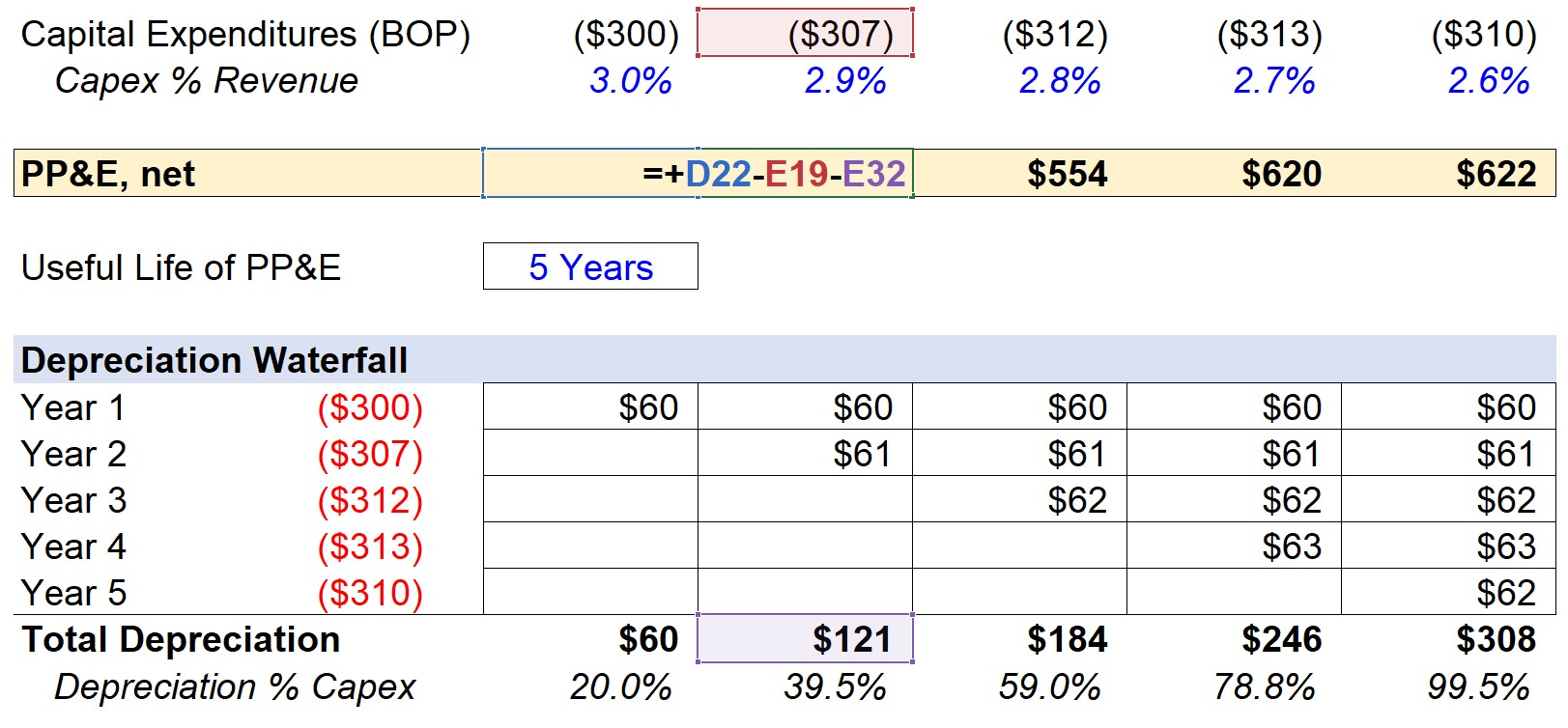## 4. Fixed Asset Roll-Forward Schedule (PP&E)

In closing, the net PP&E balance for each period is shown below in the finished model output.

While the purpose of this post was primarily to illustrate how depreciation can be forecasted, PP&E, Capex, and depreciation are three intertwined metrics that ultimately go hand-in-hand.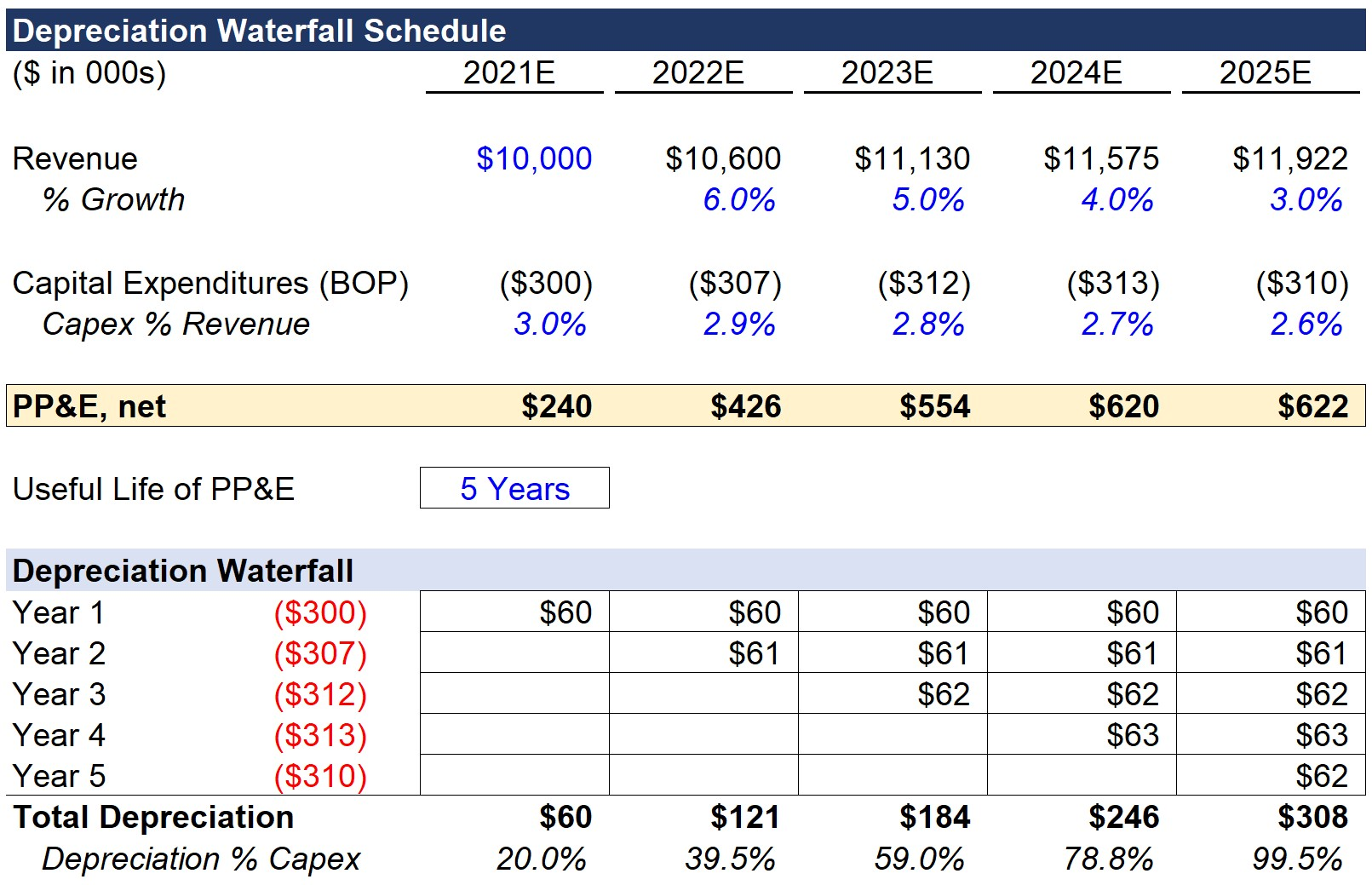Step-by-Step Online Course

### Everything You Need To Master Financial Modeling

Enroll in The Premium Package: Learn Financial Statement Modeling, DCF, M&A, LBO and Comps. The same training program used at top investment banks.Inline FeedbacksLearn Financial Modeling Online

Everything you need to master financial and valuation modeling: 3-Statement Modeling, DCF, Comps, M&A and LBO.

X

The Wall Street Prep Quicklesson Series

7 Free Financial Modeling Lessons

Get instant access to video lessons taught by experienced investment bankers. Learn financial statement modeling, DCF, M&A, LBO, Comps and Excel shortcuts.